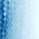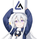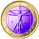# Filter Amplitude Response Estimator - A Simple Calculation

1371 görüntülenme
In digital signal processing knowing how a system interact with the frequency content of an input signal is extremely important, the mathematical tool that give you this information is called "frequency response". The frequency response regroup two elements, the amplitude response, and the phase response. The amplitude response tells you how the system modify the amplitude of the frequency components in the input signal, the phase response tells you how the system modify the phase of the frequency components in the signal, each being a function of the frequency.

The today proposed tool aim to give a low resolution representation of the amplitude response of any filter.

What Is The Amplitude Response Of A Filter ?

Remember that filters allow to interact with the frequency content of a signal by amplifying, attenuating and/or removing certain frequency components in the input signal, the amplitude (also called magnitude) response of a filter let you know exactly how your filter change the amplitude of the frequency components in the input signal, another way to see the amplitude response is as a tool that tell you what is the peak amplitude of a filter using a sinusoid of a certain frequency as input signal.

For example if the amplitude response of a filter give you a value of 0.9 at frequency 0.5, it means that the filter peak amplitude using a sinusoid of frequency 0.5 is equal to 0.9.

There are several ways to calculate the frequency response of a filter, when our filter is a FIR filter (the filter impulse response is finite), the frequency response of the filter is the absolute value of the discrete Fourier transform ( DFT ) of the filter impulse response.

If you are curious about this process, know that the DFT of a N samples signal return N values, so if our FIR filter coefficients are composed of only 5 values we would get a frequency response of 5 values...which would not be useful, this is why we "pad" our coefficients with zeros, that is we add zeros to the start and end of our series of coefficients, this process is called "zero-padding", so if our series of coefficients is: (1,2,3,4,5), applying zero padding would give (0,0...1,2,3,4,5,...0,0) while keeping a certain symmetry. This is related to the concept of resolution, a low resolution amplitude response would be composed of a low number of values and would not be useful, this is why we use zero-padding to add more values thus increasing the resolution.

Making a Fourier transform in Pinescript is not doable, as you need the complex number i for computing a DFT , but thats not even the only problem, a DFT would not be that useful anyway (as the processes to make it useful in a trading context would be way too complex). So how can we calculate a filter amplitude response without using a DFT ? The simple answer is by taking the peak amplitude of a filter using a sinusoid of a certain frequency as input, this is what the proposed tool do.

Using The Tool

The proposed tool give you a 50 point amplitude response from frequency 0.005 to 0.25 by default. the setting "Range Divisor" allow you to see the amplitude response by using a different range of frequency, for example if the range divisor is equal to 2 the filter amplitude response will be evaluated from frequency 0.0025 to 0.125.

In the script, filt hold the filter you want to see the frequency response, by default a simple moving average .

The position of the frequency response is defined by the "Show Amplitude Response At Bar Number" setting, if you want the frequency response to start at bar number 5000 then enter 5000, by default 10000. If you are not a premium set the number at 4000 and it should work.

amplitude response of a simple moving average of period 14, res = 2.

By default the amplitude response use an amplitude scale, a value of 1 represent an unchanged amplitude. You can use Dbfs (decibel full scale) instead by checking the "To Decibels (Full Scale)" setting.

Dbfs amplitude response, a value of 0 represent an unchanged amplitude.

Some Amplitude Responses

In order to prove the accuracy of the proposed tool we can compare the amplitude response given by the proposed tool with the mathematical function of the amplitude response of a simple moving average , that is:

abs(sin(pi*f*length)/(length*sin(pi*f)))

In cyan the amplitude response given by the proposed tool and in blue the above function. Below are the amplitude responses of some moving averages with period 14.

Amplitude response of an EMA , the EMA is a IIR filter, therefore the amplitude response can't be made by taking the DFT of the impulse response (as this ones has infinite length), however our tool can give its frequency response.

Amplitude response of the Hull MA, as you can see some frequencies are amplified, this is common with low-lag filters.

Gaussian moving average ( ALMA ), with offset = 0.5 and sigma = 6.

Simple moving average high-pass filter amplitude response

Center of gravity bandpass filter amplitude response

Center of gravity bandreject filter.

IMPORTANT!: The amplitude response of adaptive moving averages is not stationary and might change over time.

Conclusion

A tool giving the amplitude response of any filter has been presented, of course this method is not efficient at all and has a low resolution of 50 points (the common resolution is of 512 points) and is difficult to work with, but has the merit to work on Tradingview and can give the frequency response of IIR filters, if you really need to see the frequency response of a filter then i recommend you to use the function freqz from the scipy package.

I still hope you will enjoy using this tool to have a look at the amplitude responses of your favorite moving averages.

I'am aware of the current situation, however i'am somehow feeling left out from the pinescript community, let me know via PM if i have done something to you and i'll do my best to fix any problems i might have caused (or i might be being parano xD )

Patreon: https://www.patreon.com/alexgrover

You can also check out some of the indicators I made for luxalgo : https://www.tradingview.com/u/LuxAlgo/#published-scripts

## YorumlarYou never failed to amaze me. You certainly are one of the most innovative pine coders out there. Fantastic work!!
Cevap GönderDeVisionCap
@DeVisionCap, Thanks for these kind words, i can't tell if i'am one of the most innovative but i'am sure trying to propose novel indicators every time i post something :)
Cevap Gönder